Basics of Geometry
edHelper subscribers - Create a new printable

Number of Keys
 Select the number of different printables: 1 key 2 keys 3 keys 4 keys 5 keys

Number of Pages (for each key)
 Select the number of pages: 1 page 2 pages 3 pages 4 pages

Include an answer key (answer keys will be at the end of the printable)
High School Geometry
High School Geometry
Math

 Name _____________________________Date ___________________ (Key 1 - Answer ID # 1026695)
1.   What is the supplement of a thirty-two degree angle?
2.   What is the complement of a forty-nine degree angle?
3.   Two angles are ___________ if the sum of their measures is one hundred eighty.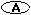complementary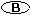adjacent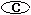supplementary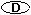congruent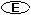obtuse angles
4.   How could you write that angle LSC is forty degrees?m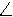LSC = 40LSC = 40LSC = 40mLSC = 40None of the above
5.   Two angles are ___________ if the sum of their measures is ninety.supplementaryright anglescongruentcomplementaryadjacent
6.   What instrument can you use to find the measure, in degrees, of an angle?scissorcalculatorprotractorcompassruler
7.   This type of angle has a measure of 180 degrees.right angleacute angleobtuse anglestraight angle
8.   IfR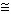J andJH thenRH is true because of what property of angle congruence?symmetricreflexivetransitive
9.   This type of angle has a measure of 90 degrees.straight angleright angleobtuse angleacute angle
10.   If two lines are perpendicular, then they form ___________.congruent non-adjacent anglesparallelcongruent adjacent anglescomplementary

Basics of Geometry
edHelper subscribers - Create a new printable

Number of Keys
 Select the number of different printables: 1 key 2 keys 3 keys 4 keys 5 keys

Number of Pages (for each key)
 Select the number of pages: 1 page 2 pages 3 pages 4 pages